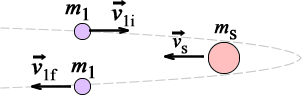8.01SC | Fall 2016 | Undergraduate

# Classical Mechanics

Week 9: Collision Theory

## 27.5 Worked Example - Gravitational Slingshot

« Previous | Next »A spacecraft of mass $$m1$$ with speed $$v_{1i} = 3v_s$$, approaches Saturn which is moving in the opposite direction with speed $$v_s$$. After interacting gravitationally with Saturn, the spacecraft swings around Saturn and heads off in the opposite direction from its approach direction. The mass of Saturn is $$m_s$$, is much much larger than the mass of the spacecraft. Find the final speed, $$v_{1f}$$, of the spacecraft after it is far enough away from Saturn to be nearly free of the gravitational pull of Saturn.

« Previous | Next »

Fall 2016
Lecture Videos
Problem Sets
Online Textbook